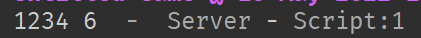# Shortening if statements by alot

``````if hit.Name == "hitbox" then
print('deleted')
print(hit.Name)
hit.Parent:Destroy()
``````

so i have this this where it assigns number hitbox like hitbox1 hitbox2
and im wondering how could i just check the number instead of if hitbox1 if hitbox2 if hitbox3 and so on

can you clarify your problem more?

heres a solution if im thinking your problem right:

`````` if string.gsub(hit.Name, "%D", "") == 1 then
--run
end
``````
``````local db = false

script.Parent.Touched:Connect(function(hit)
local str = string.gsub(hit.Name, "hitbox", "")
print("hitbox" ..str)
if db == false then
db = true
if hit.Name == "hitbox" ..str then
print('deleted')
print(hit.Name)
hit.Parent:Destroy()
end
end
wait(1)
db = false
end)
``````

this is what i’ve tried but the string is just printing as hitbox(number) instead of removing hitbox

try this then?

`````` if string.gsub(hit.Name, "%D", "") == 1 then
--run
end
``````

so basically it removes all the string except for numbers from a string

what is %D???

im trying to remove the hitbox part not the numbers

yeah i know, try it, i cant seem to find the %D in the wiki, just the %d which is for digits (numbers)

no what the script you sent would be doing is getting the hit.name (which is hitbox1234) and finding the numbers and getting rid of them. what im trying to do is get the numbers

but based on the source of where i found it,
what “%D” does is get all the characters that is not a digit,
and using string.gsub we can remove all that characters that isnt a digit and change them into the last parameter of the string.gsub

``````local hitboxNo = print(string.gsub("hitbox1234", "%D", ""))
print(hitboxNo)  -- 1234
``````

ive tested it in studio and it works fine as what you wanted.

for me it prints 1234 6 with a space between 4 and 6oh wait thats odd. i just tested it again

OHHH wait it works fine, the “6” tells how many strings were changed, and in our case its “hitbox” which contains 6 letters.

wait so when im scripting will the 6 be included or excluded

ahahaha waitt im so dumb- remove the print in the hitboxNo

``````local hitboxNo = string.gsub("hitbox1234", "%D", "")
print(hitboxNo)
``````

try this now itll work fine ahaha

ok bro!!!

1 Like